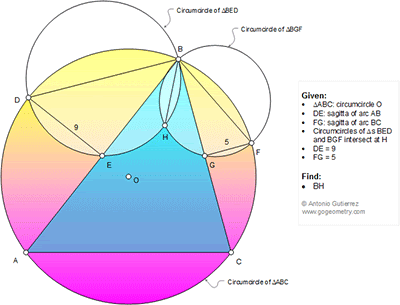## Thursday, October 29, 2015

### Geometry Problem 1159: Triangle, Circumcircle, Sagitta, Chord, Arc, Metric Relations

Level: Mathematics Education, High School, Honors Geometry, College.

Click the diagram below for more details.1.Join B to O Fröm pythagora theorem r2 = 3/2 sq2R (tr BEO and BDE) The same r3 = 1/2sq10R
From similar tr BDE and BHF we get BH = 3sq5

2.http://s29.postimg.org/8hhggawgn/pro_1159.png

Let R= radius of circle O
We have ∡ (BHD)= ∡ (BHF)=90 => D, H, F are collinear
DB^2= DẸ 2R = 18.R
BF^2= FG. 2R= 10.R
DB/ BF= sqrt(18/10)= sqrt(1.8)
Observe that F is the midpoint of arc BC so
∡ (CBF)= ∡ (BDF)= angle A/2
So triangle BDH simillar to FBG
DB/BF= BH/FG = sqrt(1.8)
BH= 5 x sqrt(1.8)= 3.sqrt(5)

3.Tr.s BDE and BHF are similar so

BD/BF = 9/BH ....(1)

Tr. s BDH and BGF are similar so

BD/BF = BH/5 ....(2)

From (1) and (2),

9/BH = BH /5

Hence BH = 3sqrt5

Sumith Peiris
Moratuwa
Sri Lanka